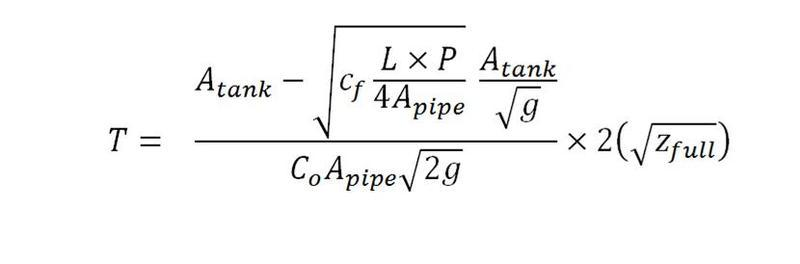# Hardcore re-arrangement help

if anyone can do this i will be very happy ive had a good old try and i end up just lost in variables
i would like to re-arrange to make apipe the subjecti ended up with the sqrt of one apipe which i dont know how to solve;

thanks

Last edited:

chiro
if anyone can do this i will be very happy ive had a good old try and i end up just lost in variables
i would like to re-arrange to make apipe the subject

View attachment 43400

i ended up with the sqrt of one apipe which i dont know how to solve;

thanks

Hey 3123marriott and welcome to the forums.

[EDIT] I made a really stupid mistake by not reading the whole thing properly so I will post reply later.

chiro
Ok then.

For this problem I think it may actually be an implicit problem meaning that you can't just represent it in a normal functional manner (like APipe(blah) = blah). This means that you will end up getting an implicit equation instead of an explicit equation. If this is the case you will have to use a root finding algorithm to get answers given that you have values for the other parameters.

It would be helpful if you show your work so that we can give you hints and help you understand for yourself what is going on.

HallsofIvy
I'd try the following (if there is no gross mistake in what follows): multiply up and down the right-hand side by ${\sqrt g}$, and pass $g \, C_0 A_{pipe} \sqrt 2$ multiplying to the left; then take $A_{tank} \sqrt{Z_{full}}$ as common factors on the right and pass them dividing to the left:$$\frac {g T C_0 A_{pipe}} {A_{tank}} \sqrt {\frac 2 {Z_{full}}} = 2 \sqrt g - \sqrt{\frac{C_f L P}{A_{pipe}}}$$Now substitute $B^2 = A_{pipe}$, and multiply through by one more $B$:$$\frac {g T C_0} {A_{tank}} \sqrt {\frac 2 {Z_{full}}} B^3 - 2 \sqrt g B + \sqrt{C_f L P} = 0$$
That's a cubic equation in $B$, which should have at least one real solution (or maybe three):
For each non-negative solution, take $A_{pipe} = \sqrt B$ as a solution to your original problem.